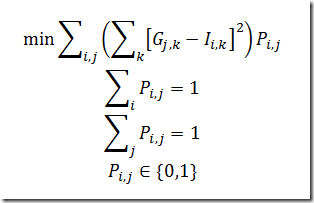## Friday, January 15, 2010

### Difficult MIQP

I would like to minimize || PG-I || over P, where P is a p x p permutation matrix
(obtained by permuting the rows and/or columns of the identity matrix), G is a
given p x p matrix with full rank and I the identity matrix.
||.|| is the frobenius norm.

This can be implemented as a MIQP:

set i /i1*i25/;
alias (i,j,k);

parameter G(i,j);
G(i,j) = uniform(-1,1);

parameter Id(i,j);
Id(i,i) = 1;

binary variable p(i,j);
variable z,v(i,j);

equation
defv(i,j)
norm
assign1(i)
assign2(j)
;

* Frobenius norm: http://mathworld.wolfram.com/FrobeniusNorm.html
* we can drop the sqrt as this is a monotonic increasing function
norm..  z =e=
sum((i,j), sqr(v(i,j)));

* we can substitute out v if needed
defv(i,j).. v(i,j) =e=
sum(k, p(i,k)*g(k,j)) - Id(i,j);

* these assignment constraints make sure P is a permutation matrix
assign1(i)..
sum(j, p(i,j)) =e= 1;
assign2(j)..
sum(i, p(i,j)) =e= 1;

model m /all/;
solve m minimizing z using miqcp;

This is not very easy to solve to optimality if set i is larger than 20. The bounds just move too slowly. When running the problem for 1000 seconds with Cplex I see:

Although new solutions are found during the search even close to the end of the run, they only show marginal improvements.

Someone suggested to solve this as an assignment problem. I don’t believe that is actually 100% correct, but the approximation turns out to be very good. The GAMS code is:

parameter c(i,j);
c(i,j) = sqrt(
sum(k, sqr(Id(i,k)-G(j,k))));

equation cassign;
cassign.. z =e=
sum((i,j),c(i,j)*p(i,j));

model assign/assign1,assign2,cassign/;
solve assign minimizing z using rmip;

Here is small example that shows some differences:

set i /i1*i6/;
alias (i,j,k);

parameter G(i,j);
*G(i,j) = uniform(0,2);
G(i,j) = normal(0,2);

parameter Id(i,j);
Id(i,i) = 1;

binary variable p(i,j);
variable z,v(i,j);

equation
defv(i,j)
norm
assign1(i)
assign2(j)
;

* Frobenius norm: http://mathworld.wolfram.com/FrobeniusNorm.html
* we can drop the sqrt as this is a monotonic increasing function
norm..  z =e=
sum((i,j), sqr(v(i,j)));

* we can substitute out v if needed
defv(i,j).. v(i,j) =e=
sum(k, p(i,k)*g(k,j)) - Id(i,j);

* these assignment constraints make sure P is a permutation matrix
assign1(i)..
sum(j, p(i,j)) =e= 1;
assign2(j)..
sum(i, p(i,j)) =e= 1;

model m /all/;
option optcr=0;
solve m minimizing z using miqcp;

scalar frobnorm1; frobnorm1 = sqrt(z.l);
option p:0;
display p.l,frobnorm1;

parameter c(i,j);
c(i,j) = sqrt(
sum(k, sqr(Id(i,k)-G(j,k))));

equation cassign;
cassign.. z =e=
sum((i,j),c(i,j)*p(i,j));

model assign/assign1,assign2,cassign/;
solve assign minimizing z using rmip;

scalar frobnorm2,diff;
frobnorm2=sqrt(
sum((i,j),sqr(sum(k, p.l(i,k)*g(k,j)) - Id(i,j))));
diff = frobnorm2-frobnorm1;
display p.l,frobnorm2,frobnorm1,diff;

The differences are:

 ----     81 PARAMETER frobnorm2            =       10.584              PARAMETER frobnorm1            =       10.502              PARAMETER diff                 =        0.082

A different suggestion seems to actually work. When we use

c(i,j) = sum(k, sqr(Id(i,k)-G(j,k)));

the results with some random G matrices are the same. So to understand this we need to show thatgive the same solutions.